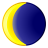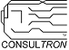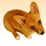# PSoC™ 5, 3 & 1 Forum DiscussionsLevel 2
Level 2# Problem getting Temp. PT100

Hello,

I tried to measure the Temperautre, but the room temperature is shown 85°C instead of 25,20°C . I don't know where the Problem could be.

Best Regards

Tarik

1 SolutionLevel 7
Level 7# Re: Problem getting Temp. PT100

I did a simple step-by-step test for  R_referense  = Rx = 100 Ohm:

at line vRef = MeasureRefResVoltage ();

result = 80 mV (LCD_Position (0,0); LCD_PrintU32Number (ADC_DelSig_CountsTo_uVolts (vRef));)

should be 100mV but this is acceptable.

at line rtdRes = GetRTDRes (vRef);

result = 124500 this corresponds to 63.3 degrees and I see it on the display (RTD Temp = 63.30).

There seems to be a problem in the int32 function GetRTDRes (int32 vRef)

possibly function zeroCurrentOffset = GetOffset (); gives the wrong result.

zeroCurrentOffset = 1300 but should be around 13000.

A similar problem was solved in this thread: Calculating RTD sensors

4 RepliesLevel 9
Level 9# Re: Problem getting Temp. PT100

Tarik,

• Why did you flip the + and - outputs of the "MUX" to the - and + inputs of the "ADC_DelSig"?  It shouldn't be a big issue, but you should receive a negative value.
• Have you confirmed that the A, B and C coefficients of your RTD calc component match your PT100 sensor?

Based on a standard PT100 table, the resistance difference between 25C and 85C is 23 ohms.  This is a significant error.

Len

Len
"Engineering is an Art. The Art of Compromise."Level 7
Level 7# Re: Problem getting Temp. PT100

I did a simple step-by-step test for  R_referense  = Rx = 100 Ohm:

at line vRef = MeasureRefResVoltage ();

result = 80 mV (LCD_Position (0,0); LCD_PrintU32Number (ADC_DelSig_CountsTo_uVolts (vRef));)

should be 100mV but this is acceptable.

at line rtdRes = GetRTDRes (vRef);

result = 124500 this corresponds to 63.3 degrees and I see it on the display (RTD Temp = 63.30).

There seems to be a problem in the int32 function GetRTDRes (int32 vRef)

possibly function zeroCurrentOffset = GetOffset (); gives the wrong result.

zeroCurrentOffset = 1300 but should be around 13000.

A similar problem was solved in this thread: Calculating RTD sensorsLevel 7
Level 7# Re: Problem getting Temp. PT100

Try setting a delay for DAC current stabilization  in these 3 functions:

int32 MeasureRefResVoltage()

....

/* Configure the IDAC to source 1 mA */

IDAC_SetValue(125);

CyDelay(50);

....

int32 GetRTDRes(int32 vRef)

....

/* Set the IDAC to pass 1 mA */

IDAC_SetValue(125);

CyDelay(50);

....

int32 GetOffset(void)

....

IDAC_SetValue(1);

CyDelay(50);

....Level 8
Level 8# Re: Problem getting Temp. PT100